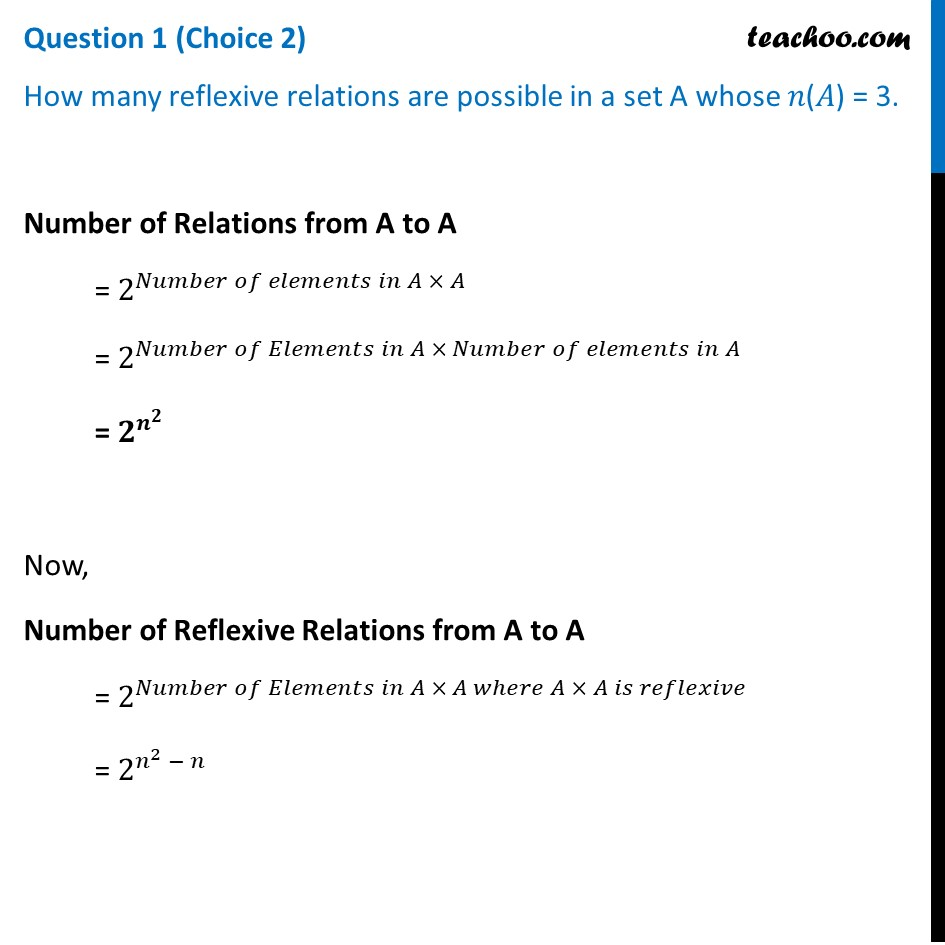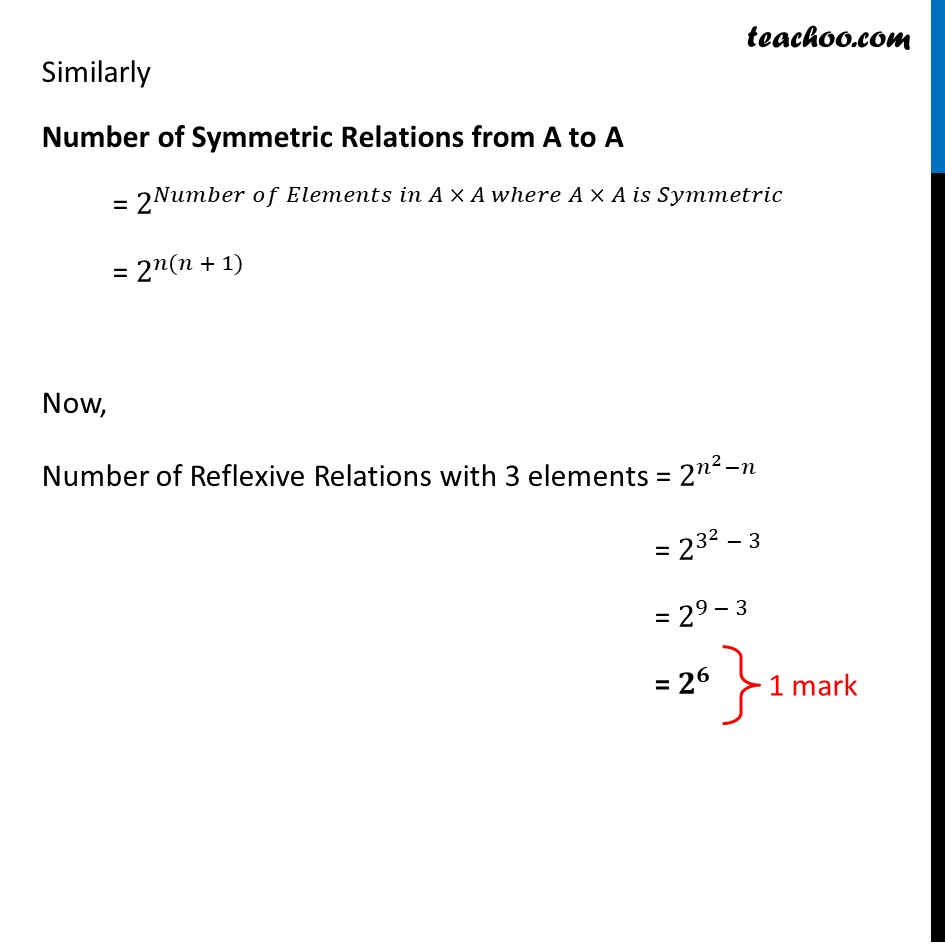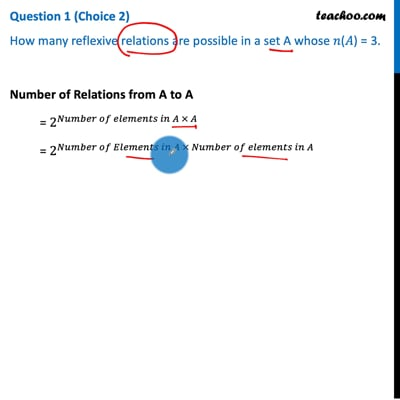CBSE Class 12 Sample Paper for 2021 Boards

Class 12
Solutions of Sample Papers and Past Year Papers - for Class 12 Boards

## How many reflexive relations are possible in a set A whose 𝑛(𝐴) = 3.This video is only available for Teachoo black users

Introducing your new favourite teacher - Teachoo Black, at only ₹83 per month

### Transcript

Question 1 (Choice 2) How many reflexive relations are possible in a set A whose 𝑛(𝐴) = 3. Number of Relations from A to A = 2^(𝑁𝑢𝑚𝑏𝑒𝑟 𝑜𝑓 𝑒𝑙𝑒𝑚𝑒𝑛𝑡𝑠 𝑖𝑛 𝐴 × 𝐴) = 2^(𝑁𝑢𝑚𝑏𝑒𝑟 𝑜𝑓 𝐸𝑙𝑒𝑚𝑒𝑛𝑡𝑠 𝑖𝑛 𝐴 × 𝑁𝑢𝑚𝑏𝑒𝑟 𝑜𝑓 𝑒𝑙𝑒𝑚𝑒𝑛𝑡𝑠 𝑖𝑛 𝐴) = 𝟐^(𝒏^𝟐 ) Now, Number of Reflexive Relations from A to A = 2^(𝑁𝑢𝑚𝑏𝑒𝑟 𝑜𝑓 𝐸𝑙𝑒𝑚𝑒𝑛𝑡𝑠 𝑖𝑛 𝐴 × 𝐴 𝑤ℎ𝑒𝑟𝑒 𝐴 × 𝐴 𝑖𝑠 𝑟𝑒𝑓𝑙𝑒𝑥𝑖𝑣𝑒) = 2^(𝑛^2 − 𝑛) Similarly Number of Symmetric Relations from A to A = 2^(𝑁𝑢𝑚𝑏𝑒𝑟 𝑜𝑓 𝐸𝑙𝑒𝑚𝑒𝑛𝑡𝑠 𝑖𝑛 𝐴 × 𝐴 𝑤ℎ𝑒𝑟𝑒 𝐴 × 𝐴 𝑖𝑠 𝑆𝑦𝑚𝑚𝑒𝑡𝑟𝑖𝑐) = 2^(𝑛(𝑛 + 1)) Now, Number of Reflexive Relations with 3 elements = 2^(𝑛^2−𝑛) = 2^(3^2 − 3) = 2^(9 − 3) = 𝟐^𝟔